# Improved Fixed Point Methods for Loss Networks with Linear Structure

M.S. Bebbington, P.K. Pollettand I. ZiedinsExtended abstract

This paper is concerned with the performance evaluation of loss networks. For the simplest networks, there are explicit analytical formulae for the important measures of performance, but for networks which involve some level of dynamic control, such as routing schemes which make use of spare capacity, exact analytical methods have had only limited success. Under several regimes, the Erlang Fixed Point (EFP) method provides a good approximation for the blocking probabilities, but when these regimes are not operative, the method can perform badly because the key assumption of independent blocking may not hold. We propose several new models which specifically account for the dependencies between neighbouring links. We derive approximations for the blocking probabilities with errors typically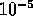of that found using the EFP approximation. These are extended to cases where admission controls, such as trunk reservation, are used.

We will focus attention on simple, highly linear networks, since here the EFP approximation is expected to perform poorly. To illustrate our methods, consider a loss network with K links forming a loop and each link has the same capacity, C. There are two types of traffic: 1-link routes (type-1 traffic) and 2-link routes comprising pairs of adjacent links (type-2 traffic). Type-t traffic is offered at rateon each type-t route. Ifis the common loss probability for type-t calls, then the EFP approximation iswhere the Erlang Fixed Point B is the unique solution towhere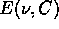is Erlang's Formula.

A significant improvement in accuracy can be obtained by adapting methods of Pallant . The network is decomposed into independent subnetworks and the stationary distribution is evaluated for each. Consider a two-link subnetwork consisting of say links 1 and 2. There are three routes:,and. If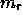denotes the number of calls on route r, thenis the number of calls occupying capacity on link 1 but not on link 2,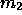is the number occupying capacity on link 2 but not on link 1, and,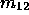is the number of calls occupying capacity on both links. The state space for the subnetwork is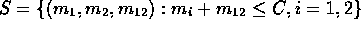and the stationary distribution iswhere G is a normalizing constant; we estimate B, the probability that a link adjacent to the two-link subnetwork is fully occupied, by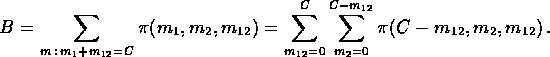These expressions are used iteratively to determine a fixed point B and then, as before, we setand.

Our second, and most accurate, approximation uses additional knowledge of the state of a given link in estimating the probability that the adjacent link is full. We use state-dependent arrival rates,,, whereis the probability that link K is fully occupied, conditional on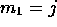(is also the probability that link 3 is fully occupied, conditional on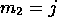), so thatOnceis estimated anddetermined, we set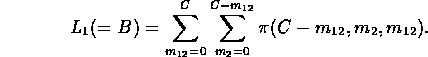Similarly,can be expressed in terms of. An estimate ofis found by assuming thatdoes not depend on. For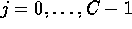, we setwhereThe dependence of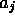onis due to the cyclic nature of the network, but is expected to be slight for large networks.

Full details of all fixed point methods will be described in the paper, including extensions to networks with trunk reservation. Detailed comparisons and error analysis will be provided for all methods.